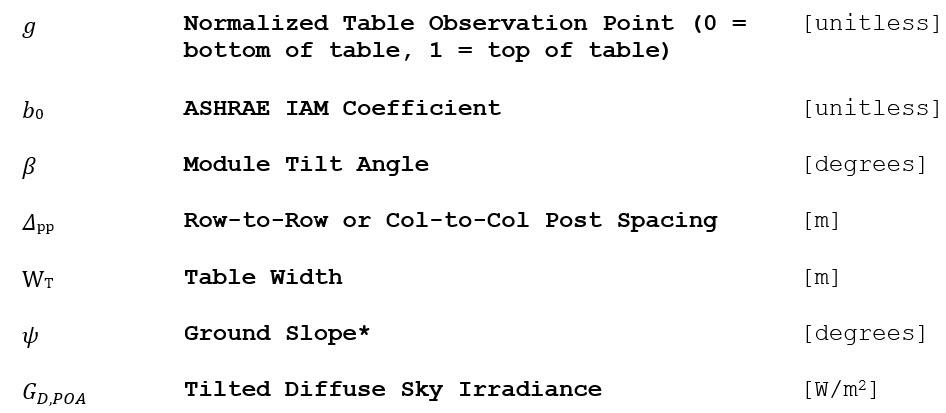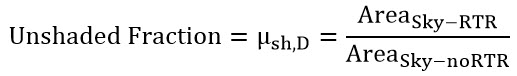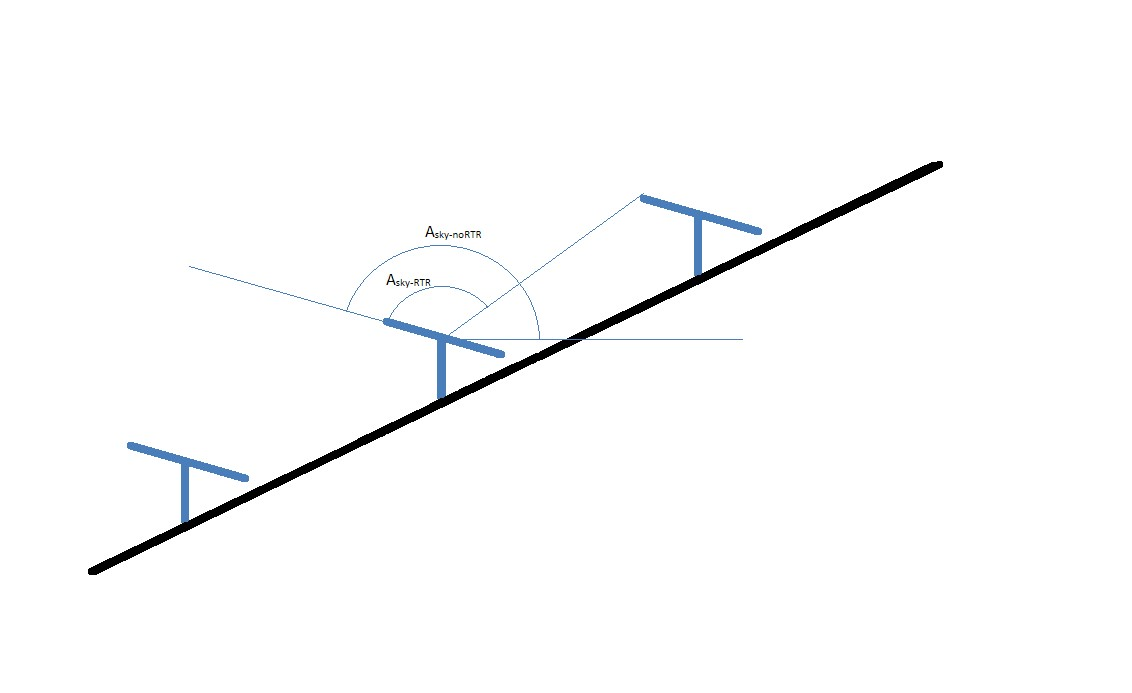Loss of irradiance due to shading is an important calculation for fixed tilt and tracker PV power plants. In addition to modeling direct beam shading, PlantPredict models diffuse shading. Diffuse shading is modeled using a diffuse sky-shading factor, which represents the effective fraction of sky dome lost due to near objects. These calculations are currently restricted to row-to-row shading.

The diffuse shading algorithm used by PlantPredict assumes a clear sky isotropic sky dome. It also assumes that rows of modules have infinite length. Diffuse shading is calculated for the midpoint of the table, represented by Point A in Figure 23, which is deemed representative for the entire table.## Inputs*Found using equations from Near Direct Beam Shading

## Outputs## Algorithm

To estimate near diffuse shading factor, the portion of the sky dome visible to Point A is determined with and without the preceding row of modules. As seen in the equation below, the ratio of the former to the latter is the near diffuse shading factor (i.e. the unshaded fraction).AreaSky-RTR is the fraction of the sky visible to point A after row-to-row shading. It is not the area of the sky lost due to row-to-row shading. AreaSky-noRTR is the fraction of the sky dome that is visible to point A if the preceding row of modules was not present. Please note that, unless the module tilt = 0°, the denominator of the equation above is not equal to the full sky dome. This is because some of the sky dome is lost to the ground.  The geometry used to define both AreaSky-RTR and AreaSky-noRTR is shown in Figure 24.### Figure 24. Geometry definitions for diffuse shading model.

In Figure 24, β is equal to the module tilt. γ is the angle between the horizontal running through point A and the line connecting point A to point C (the top of the preceding row of modules). α is the angle between the surface normal from point A and the line connecting point A to point C.

Angles are defined as:The final equation for AreaSky-RTR is shown as:Where Atop = tan (β + γ).

Because of the periodicity of the tangent function, if α < 0 then AreaSky-RTR actually becomes the shaded area of the sky, as opposed to the visible portion of the sky, and the following adjustment is made to the output of the equation for AreaSky-RTR.The final equation for AreaSky-noRTR is nearly identical to that used for AreaSky-RTR and is included below.Where Aβ = tan (β).

Note that the equations above include the ASHRAE incidence angle modifier b0. PlantPredict uses the same equations to compute diffuse shading and diffuse incidence angle losses. When computing diffuse shading losses, b0 is assumed to be zero. When computing the diffuse incidence angle modifier, μD,IAM, the equation below is used.The effective sky diffuse irradiance on the tilted plane after diffuse IAM and shading, GD,Shd,IAM, is shown as:In the above equation, GD,POA is tilted diffuse sky irradiance.

For a sloped array, the function is changed such that γ is now a function of the ground slope.The diffuse shading algorithm accounts for ground slope that would place one row of modules higher, or lower, than the proceeding row. However, it does not currently account for ground slope along the length of rows. Examples of ground slope shading modeled by PlantPredict are shown in Figure 25 through Figure 27.

###Figure 25: Downward sloping ground, when shading is caused by the preceding row.### Figure 26: Downward sloping ground, when shading is caused by the subsequent row.